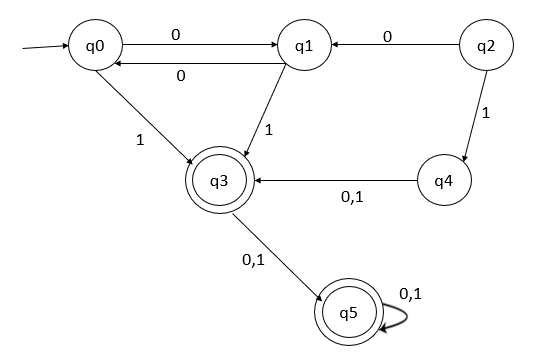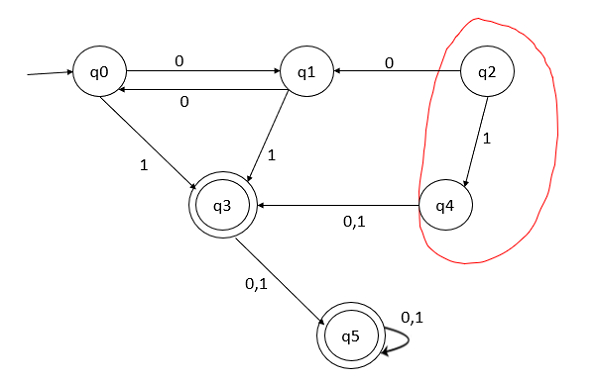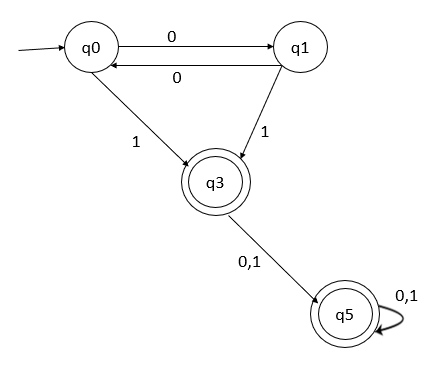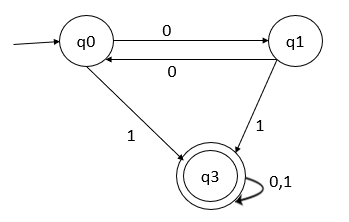# What is Minimization of DFA?

Data Structure AlgorithmsComputer ScienceComputers

## Problem

Given a Deterministic Finite Automata (DFA), try to reduce the DFA by removing unreachable states and removing similar rows.## Solution

### Step 1

Remove the unreachable states from q0

From the initial states, we are not able to reach q2 and q4. So, remove these two states as shown below −After removing unreachable states, the partial minimized DFA is as follows −### Step 2

The transition table is given below −

States01
->q0q1q3
q1q0q3
*q3q5q5
*q5q5q5

### Step 3

Divide tables into 2 tables as shown below −

Table 1 starts from the non-final states.

States01
->q0q1q3
q1q0q3

Table 2 starts from the final states.

States01
*q3q5q5
*q5q5q5

### Step 4

Remove similar rows.

Table 1 has no similar rows

Table 2 has similar rows. So, skip q5 and replace q5 by q3

States01
q3q5q3

### Step 5

Combine two tables as shown below −

States01
->q0q1q3
q1q0q3
*q3q3q3

Thus, the minimized DFA will be as follows −